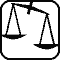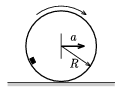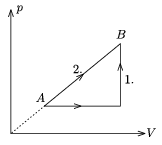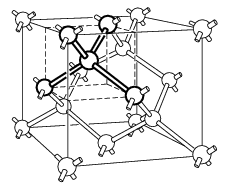Mathematical and Physical Journal
for High Schools
Issued by the MATFUND Foundation
 Already signed up? New to KöMaL?

# Exercises and problems in PhysicsMarch 2003## Experimental problem

M. 241. Place onto each other two optical gratings of the same groove spacing (grating constant) with their grooves including an angle $\displaystyle \varphi$. Light the gratings with laser beam or white light, make an image of a tiny hole on the screen, then put the two gratings before or after an imaging objective lens. What shall we see on the screen with different $\displaystyle \varphi$ angles (e.g. 0o, 30o, 45o, 60o, 90o)?

(6 points)## Theoretical problems

It is allowed to send solutions for any number of problems, but final scores of students of grades 9-12 are computed from the 5 best score in each month. Final scores of students of grades 1-8 are computed from the 3 best scores in each month.

P. 3601. The elements of a peculiar bead string are made of the same material. All the beads are of spherical shape and have a cylindrical borehole in their middle. The peculiarity is that though the radii of the spheres are different, the borehole length (the height of the cylinder) is exactly the same in all beads. What can we say about the masses of the beads?

(3 points)

P. 3602. There is a conical frustum shape glass, broadening towards the top. Will the pressure at the bottom be greater, when we pour water or when we pour oil of the same mass into the glass?

(3 points)

P. 3603. Inside a uniformly accelerating thin-walled sphere of radius R, which is rolling on horizontal soil, there is a small body slipping around. What friction coefficient is required to ensure the small body to stay at a R/2 distance from the soil?(4 points)

P. 3604. The Helka motorboat traveling from Fonyód to Badacsony at a speed of 20 km/h emits a sound of 100 Hz, which is reflected from a catamaran aproaching along exactly the same path as Helka goes. A sailor on the Helka notices 7 beats in a second. What is the speed of the catamaran?

(5 points)

P. 3605. A diatomic gas of a certain mass gets from state A to state B on two ways shown in the figure. The ratio of the amounts of heat absorbed in the two processes is Q2:Q1=54:49. a) Determine the ratio of the amounts of work done in the two processes. b) What is the temperature of the gas in state B if it is 100 K in state A?(4 points)

P. 3606. In a vertical, solid copper conductor cable of a radius of 2 cm leading to an electrolyzer there is a 100 A electric current. From what distance of the axis of the conductor will the size of the generated magnetic induction vector be 2.10-4 T?

(4 points)

P. 3607. What pressure is exerted by the Sun's light on an east-looking window on 22 march at 6 o'clock in the morning if the window reflects 4%, absorbs 6% of the light and lets through the rest of it. The intensity of the incoming sunlight is 1000 W/m2.

(4 points)

P. 3608. A photon of a wavelength of $\displaystyle \lambda$0 collides with an electron moving perpendicular to the photon's path. After the interaction the electron stays still and the photon's path is deflected by a $\displaystyle \vartheta$ angle. Determine the values of $\displaystyle \lambda$0 and $\displaystyle \vartheta$ if the wavelength of the scattered photon is $\displaystyle \lambda$0/2.

(5 points)

P. 3609. What will happen to the energy of a compressed spring if it dissolves in hydrochloric acid in its compressed state?

(3 points)

P. 3610. One of the biggest diamonds on Earth is of 44.5 carats and is almost pure carbon. Every carbon atom is connected to 4 others with such bond angles as the ones of which the four points of a regular tetrahedron can be seen from the tetrahedron's center. If we visualize the chemical bonds with rods connecting the atomic centers, and putting all the rods into one long line, how far would this linearly arranged chemical bond chain'' reach? Hint: The diamond structure can also be considered as a cubic face-centered lattice repeated with an offset along the body diagonal at a quarter length of the body diagonal.(4 points)